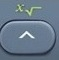# RootBasics
RootNumber

The root sign √   is also known as the radical sign.

Second Root of 49  =  ²49  =  ²√  =  ²√7 × 7 = 7
The second root is also known as the square root.

Third Root of 343  =  ³343  =  ³√  =  ³√7 × 7 × 7 = 7
The third root is also known as the cube root.

Fourth Root of 2401  =  42401  =  474  =  47 × 7 × 7 × 7 = 7

Fifth Root of 16807  =  516807  =  575  =  57 × 7 × 7 × 7 × 7 = 7is the root command.activatesabove.

Question
What is 81679616 ?

6

Calculator Click What You See Commentblinker clears screen1679616 81679616 Eighth Root6 Answer
###### Root

Question
What is 5184243.5179 ?

11.3

Calculator Click What You See Commentblinker clears screen184243.5179 5184243.5179 Fifth Root11.3 Answer
###### Root

Practice – Questions
1.  What is the fourth root of 625?

2.  What is the sixth root of 262144?

3.  What is the ninth root of 512?

4.  What is the seventh root of 37366.94531?

5.  What is the fifth root of 663382.9098?